# The MODEL Procedure

### Transformation of Error Terms

Subsections:

In PROC MODEL you can control the form of the error term. By default, the error term is assumed to be additive. This section demonstrates how to specify nonadditive error terms and discusses the effects of these transformations.

#### Models with Nonadditive Errors

The estimation methods used by PROC MODEL assume that the error terms of the equations are independently and identically distributed with zero means and finite variances. Furthermore, the methods assume that the RESID.name equation variable for normalized form equations or the EQ.name equation variable for general form equations contains an estimate of the error term of the true stochastic model whose parameters are being estimated. Details on RESID.name and EQ.name equation variables are in the section Equation Translations.

To illustrate these points, consider the common loglinear model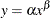(1)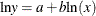(2)

where a =log() and b =. Equation (2) is called the log form of the equation in contrast to equation (1), which is called the level form of the equation. Using the SYSLIN procedure, you can estimate equation (2) by specifying

   proc syslin data=in;
model logy=logx;
run;


where LOGY and LOGX are the logs of Y and X computed in a preceding DATA step. The resulting values for INTERCEPT and LOGX correspond to a and b in equation (2).

Using the MODEL procedure, you can try to estimate the parameters in the level form (and avoid the DATA step) by specifying

   proc model data=in;
parms alpha beta;
y = alpha * x ** beta;
fit y;
run;


where ALPHA and BETA are the parameters in equation (1).

Unfortunately, at least one of the preceding is wrong; an ambiguity results because equations (1) and (2) contain no explicit error term. The SYSLIN and MODEL procedures both deal with additive errors; the residual used (the estimate of the error term in the equation) is the difference between the predicted and actual values (of LOGY for PROC SYSLIN and of Y for PROC MODEL in this example). If you perform the regressions discussed previously, PROC SYSLIN estimates equation (3) while PROC MODEL estimates equation (4).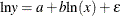(3)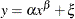(4)

These are different statistical models. Equation (3) is the log form of equation (5)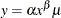(5)

where=e. Equation (4), on the other hand, cannot be linearized because the error term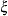(different from) is additive in the level form.

You must decide whether your model is equation (4) or (5). If the model is equation (4), you should use PROC MODEL. If you linearize equation (1) without considering the error term and apply SYSLIN to MODEL LOGY=LOGX, the results will be wrong. On the other hand, if your model is equation (5) (in practice it usually is), and you want to use PROC MODEL to estimate the parameters in the level form, you must do something to account for the multiplicative error.

PROC MODEL estimates parameters by minimizing an objective function. The objective function is computed using either the RESID.-prefixed equation variable or the EQ.-prefixed equation variable. You must make sure that these prefixed equation variables are assigned an appropriate error term. If the model has additive errors that satisfy the assumptions, nothing needs to be done. In the case of equation (5), the error is nonadditive and the equation is in normalized form, so you must alter the value of RESID.Y.

The following assigns a valid estimate ofto RESID.Y:

   y = alpha * x ** beta;
resid.y = actual.y / pred.y;


However,=e, and therefore, cannot have a mean of zero, and you cannot consistently estimateandby minimizing the sum of squares of an estimate of. Instead, you use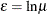.

   proc model data=in;
parms alpha beta;
y = alpha * x ** beta;
resid.y = log( actual.y / pred.y );
fit y;
run;


If the model was expressed in general form, this transformation becomes

   proc model data=in;
parms alpha beta;
EQ.trans = log( y / (alpha * x ** beta));
fit trans;
run;


Both examples produce estimates ofandof the level form that match the estimates of a and b of the log form. That is, ALPHA=exp(INTERCEPT) and BETA=LOGX, where INTERCEPT and LOGX are the PROC SYSLIN parameter estimates from the MODEL LOGY=LOGX. The standard error reported for ALPHA is different from that for the INTERCEPT in the log form.

The preceding example is not intended to suggest that loglinear models should be estimated in level form but, rather, to make the following points:

• Nonlinear transformations of equations involve the error term of the equation, and this should be taken into account when transforming models.

• The RESID.-prefixed and the EQ.-prefixed equation variables for models estimated by the MODEL procedure must represent additive errors with zero means.

• You can use assignments to RESID.-prefixed and EQ.-prefixed equation variables to transform error terms.

• Some models do not have additive errors or zero means, and many such models can be estimated using the MODEL procedure. The preceding approach applies not only to multiplicative models but to any model that can be manipulated to isolate the error term.

#### Predicted Values of Transformed Models

Nonadditive or transformed errors affect the distribution of the predicted values, as well as the estimates. For the preceding loglinear example, the MODEL procedure produces consistent parameter estimates. However, the predicted values for Y computed by PROC MODEL are not unbiased estimates of the expected values of Y, although they do estimate the conditional median Y values.

In general, the predicted values produced for a model with nonadditive errors are not unbiased estimates of the conditional means of the endogenous value. If the model can be transformed to a model with additive errors by using a monotonic transformation, the predicted values estimate the conditional medians of the endogenous variable.

For transformed models in which the biasing factor is known, you can use programming statements to correct for the bias in the predicted values as estimates of the endogenous means. In the preceding log-linear case, the predicted values are biased by the factor exp(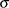/2). You can produce approximately unbiased predicted values in this case by writing the model as

   proc model data=in;
parms alpha beta;
y=alpha * x ** beta;
resid.y = log( actual.y / pred.y );
fit y;
run;


See Miller (1984) for a discussion of bias factors for predicted values of transformed models.

Note that models with transformed errors are not appropriate for Monte Carlo simulation that uses the SDATA= option. PROC MODEL computes the OUTS= matrix from the transformed RESID.-prefixed equation variables, while it uses the SDATA= matrix to generate multivariate normal errors, which are added to the predicted values. This method of computing errors is inconsistent when the equation variables have been transformed.